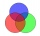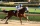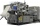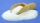# Fraction calculator

The calculator performs basic and advanced operations with fractions, expressions with fractions combined with integers, decimals, and mixed numbers. It also shows detailed step-by-step information about the fraction calculation procedure. Solve problems with two, three, or more fractions and numbers in one expression.

## Result:

### 183/4 - 57/8 = 103/8 = 12 7/8 = 12.875

Spelled result in words is one hundred three eighths (or twelve and seven eighths).

### How do you solve fractions step by step?

1. Conversion a mixed number 18 3/4 to a improper fraction: 18 3/4 = 18 3/4 = 18 · 4 + 3/4 = 72 + 3/4 = 75/4

To find new numerator:
a) Multiply the whole number 18 by the denominator 4. Whole number 18 equally 18 * 4/4 = 72/4
b) Add the answer from previous step 72 to the numerator 3. New numerator is 72 + 3 = 75
c) Write a previous answer (new numerator 75) over the denominator 4.

Eighteen and three quarters is seventy-five quarters
2. Conversion a mixed number 5 7/8 to a improper fraction: 5 7/8 = 5 7/8 = 5 · 8 + 7/8 = 40 + 7/8 = 47/8

To find new numerator:
a) Multiply the whole number 5 by the denominator 8. Whole number 5 equally 5 * 8/8 = 40/8
b) Add the answer from previous step 40 to the numerator 7. New numerator is 40 + 7 = 47
c) Write a previous answer (new numerator 47) over the denominator 8.

Five and seven eighths is forty-seven eighths
3. Subtract: 75/4 - 47/8 = 75 · 2/4 · 2 - 47/8 = 150/8 - 47/8 = 150 - 47/8 = 103/8
For adding, subtracting, and comparing fractions, it is suitable to adjust both fractions to a common (equal, identical) denominator. The common denominator you can calculate as the least common multiple of both denominators - LCM(4, 8) = 8. In practice, it is enough to find the common denominator (not necessarily the lowest) by multiplying the denominators: 4 × 8 = 32. In the next intermediate step, the fraction result cannot be further simplified by canceling.
In words - seventy-five quarters minus forty-seven eighths = one hundred three eighths.

#### Rules for expressions with fractions:

Fractions - use the slash “/” between the numerator and denominator, i.e., for five-hundredths, enter 5/100. If you are using mixed numbers, be sure to leave a single space between the whole and fraction part.
The slash separates the numerator (number above a fraction line) and denominator (number below).

Mixed numerals (mixed fractions or mixed numbers) write as non-zero integer separated by one space and fraction i.e., 1 2/3 (having the same sign). An example of a negative mixed fraction: -5 1/2.
Because slash is both signs for fraction line and division, we recommended use colon (:) as the operator of division fractions i.e., 1/2 : 3.

Decimals (decimal numbers) enter with a decimal point . and they are automatically converted to fractions - i.e. 1.45.

The colon : and slash / is the symbol of division. Can be used to divide mixed numbers 1 2/3 : 4 3/8 or can be used for write complex fractions i.e. 1/2 : 1/3.
An asterisk * or × is the symbol for multiplication.
Plus + is addition, minus sign - is subtraction and ()[] is mathematical parentheses.
The exponentiation/power symbol is ^ - for example: (7/8-4/5)^2 = (7/8-4/5)2

#### Examples:

adding fractions: 2/4 + 3/4
subtracting fractions: 2/3 - 1/2
multiplying fractions: 7/8 * 3/9
dividing Fractions: 1/2 : 3/4
exponentiation of fraction: 3/5^3
fractional exponents: 16 ^ 1/2
adding fractions and mixed numbers: 8/5 + 6 2/7
dividing integer and fraction: 5 ÷ 1/2
complex fractions: 5/8 : 2 2/3
decimal to fraction: 0.625
Fraction to Decimal: 1/4
Fraction to Percent: 1/8 %
comparing fractions: 1/4 2/3
multiplying a fraction by a whole number: 6 * 3/4
square root of a fraction: sqrt(1/16)
reducing or simplifying the fraction (simplification) - dividing the numerator and denominator of a fraction by the same non-zero number - equivalent fraction: 4/22
expression with brackets: 1/3 * (1/2 - 3 3/8)
compound fraction: 3/4 of 5/7
fractions multiple: 2/3 of 3/5
divide to find the quotient: 3/5 ÷ 2/3

The calculator follows well-known rules for order of operations. The most common mnemonics for remembering this order of operations are:
PEMDAS - Parentheses, Exponents, Multiplication, Division, Addition, Subtraction.
BEDMAS - Brackets, Exponents, Division, Multiplication, Addition, Subtraction
BODMAS - Brackets, Of or Order, Division, Multiplication, Addition, Subtraction.
GEMDAS - Grouping Symbols - brackets (){}, Exponents, Multiplication, Division, Addition, Subtraction.
Be careful, always do multiplication and division before addition and subtraction. Some operators (+ and -) and (* and /) has the same priority and then must evaluate from left to right.

## Fractions in word problems:

• Square metal sheetWe cut out four squares of 300 mm side from a square sheet metal plate with a side of 0,7 m. Express the fraction and the percentage of waste from the square metal sheet.
• SadieSadie practiced her spelling words for 3/4 of an hour, and Max practiced his spelling words for 5/12 of an hour. In the simplest form, how much longer did Sadie practice than Max?
• A laundryMr. Green washed 1/4 of his laundry. His son washed 3/7 of it. Who washed most of the laundry? How much of the laundry still needs to be washed?
• Circular gardenAlice creates a circular vegetable garden. Tomatoes are planted in 1/3 of the circular garden, carrots are planted in 2/5 of the circular garden, and green peppers are planted in 1/10 of the circular garden. What fraction represents the remaining unplante
• Coloured teacupsThe teacups in Tea Stop 55 are `2/5` green and `3/10` yellow. What fraction of the teacups are neither green nor yellow?
• Animal speciesOf 100 types of animals, 9/100 were discovered in ancient times, and 2/100 were discovered in the Middle Ages. Another 3/10 were discovered in the 1800s. What fraction of the 100 types of animals was discovered after the 1800s? Explain.
• Erika adminErika’s career consists of filing, typing and answering phones. She spends 1/6 of her time filing and 5/8 of her time typing. What fraction of her time does she spend answering phone calls?
• PizzasBilly ate 1 1/4 pizzas and John ate 1 2/3 pizzas. How much more pizza did John eat than Billy?
• Sugar 8Heather has 2 cups of powdered sugar. She sprinkles 3/5 of the sugar onto a plate of brownies and sprinkles the rest into a plate of lemon cookies. How much sugar does Heather sprinkle on the brownies? How much sugar does Heather sprinkle on the lemon coo
• Math testBrayden was solving some math problems for the math team. He answered 2 math problems. Matthew answered 3, John answered 1 reasoning. Matthew 1/2 times as many. Brayden said that 2/6. Is he correct? Why or why not? Be sure to explain your answer.
• The boyThe boy scouts spent 10/12 hour doing their daily exercises. They only used 1/4 hour in hiking. How much time did they use for other body exercises?
• Birthday partyJay brought 2 pizzas for his birthday party. At the birthday party, they eye 1 4/7 pizzas . What’s the fraction of pizzas they have left?
• The BakeryAt a Bakery, ⅗ of the baked goods are pies, and the rest are cakes. ⅓ of the pies are coconut. ⅙ of the cakes are angel food. What fraction of all the baked goods at the Bakery are coconut pies? What fraction of all the baked goods at the Bakery are are a